##The Sagnac experiment

The Sagnac experiment was sufficient proof of the inequality(and indirect evidence for the classic law of addition of velocities). Recall that four mirrors (more exactly three mirrors B and one plate H - see Fig) were installed along the periphery of a disc rotating at angular rate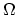.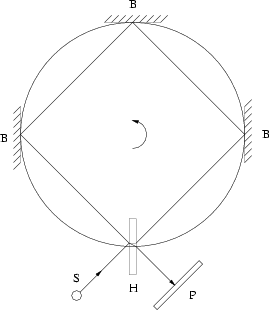A light beam was divided (by the plate H) into two beams, and one beam (1) traveled counterclockwise (in the direction of rotation) while the other traveled clockwise (2). An interference was observed at meeting of these beams. The fringe shift (as a result of the difference in times of propagation of light beams) had magnitude:.
It is obvious that the non-inertial character of the system rotating atis of no concern: nobody saw a curved light beam in vacuum; light travels between two reflections rectilinearlyIm sorry to say, but its not true. If we rotates the plate, the reflection will not be rectangular. In case of beam (1), the angle of reflection will be smaller, while with beam (2) will be greater than 90o..

Nevertheless, we consider the following mental experiment: Imagine that the disc radius tends to infinity, but the value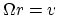remains constant. Then we have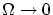. Therefore, the value of the acceleration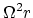tends to zero. Let us choose a radiussuch that the acceleration is much less than any pre-specified value (the existing experimental accuracy, for example). Nobody can distinguish this "near-inertial" system from a true inertial system. If the number of equidistant mirrors is also increased (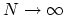), then the straight line (of light beams) between mirrors approaches the disc circle. As a result the fringe shift can be expressed as, where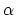is a constant for a given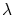andis the circumference. Because of the obvious symmetry of the experiment, the effect is additive inand its value can be related to the unit length. A "cumulative" effect of acceleration can be made less than any pre-specified value for a given straightline region. Thus, we have for the magnitude of the fringe shift:(some variations inproduce appropriate variations in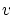, since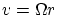is a finite value).

Therefore, the time of signal propagation linearly depends on the velocity of the motion of the system, that is Im sorry it is not true, because only equivalent with this: ray (1) perform longer path than the other (2).,.

Thats all seems to be beautiful, just a bit patch is the following: ray (1) goes on longer path compared to ray (2), until recombination. Ergo, not the speed of light was changed, merely the light (1) should perform angle 360 + gamma, and ray clockwise (2) should go only 360 - gamma, where gamma = angle of disc rotation between light emission and recombination. The speed of light always constant compared to DVAG aether.

Astrojan